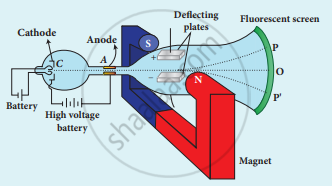Tamil Nadu Board of Secondary EducationHSC Science Class 12th

# Explain the J. J. Thomson experiment to determine the specific charge of electron. - Physics

Explain the J. J. Thomson experiment to determine the specific charge of an electron.

#### Solution

1. In 1887, J.J. Thomson measured the specific charge (e/m) of electron.
2. The specific charge is defined as the charge per unit mass of the particle.
Principle:
3. In the presence of electric and magnetic fields, the cathode rays are deflected.Arrangement of J.J. Thomson experiment to determine the specific charge of an electron
4. A highly evacuated discharge tube is used and cathode rays (electron beam) produced at the cathode are attracted towards anode disc A.
5. An anode disc is made with pin hole in order to allow only a narrow beam of cathode rays.
6. These cathode rays are now allowed to pass through the parallel metal plates, maintained at high voltage.
7. Further, this gas discharge tube is kept in between pole pieces of magnet such that both electric and magnetic fields are perpendicular to each other.
8. When the cathode rays strike the screen, they produce scintillation and hence bright spot is observed.
9. This is achieved by coating the screen with zinc sulphide.
• Determination of velocity of cathode rays:
1. For a fixed electric field between the plates, the magnetic field is adjusted such that the cathode rays (electron beam) strike at the original position O
2. i.e the magnitude of electric force is balanced by the magnitude of force due to the magnetic field.
eE = eBν
=> ν = "E"/"B"    ...(1)
e- charge of the cathode rays
3. Electric force balances the magnetic force – the path of the electron beam is a straight line.

• Determination of specific charge:
1. Since the cathode rays (electron beam) are accelerated from cathode to anode, the potential energy of the electron beam at the cathode is converted into kinetic energy of the electron beam at the anode.
2. Let V be the potential difference between anode and cathode, then the potential energy is eV.
3. Then from law of conservation of energy.
eV = 1/2 "mv"^2
=> "e"/"m" = 1/"2V"  "v"^2
4. Substituting the value of velocity from equation (1), we get
"e"/"m" = 1/"2V" "E"^2/"B"^2
5. Substituting the values of E, B and V, the specific charge can be determined as
"e"/"m" = 1.7 xx 10^11 C kg-1.
Concept: Introduction to Atom Models
Is there an error in this question or solution?
Chapter 9: Atomic and Nuclear physics - Evaluation [Page 191]

#### APPEARS IN

Tamil Nadu Board Samacheer Kalvi Class 12th Physics Volume 1 and 2 Answers Guide
Chapter 9 Atomic and Nuclear physics
Evaluation | Q III. 1. | Page 191
Share